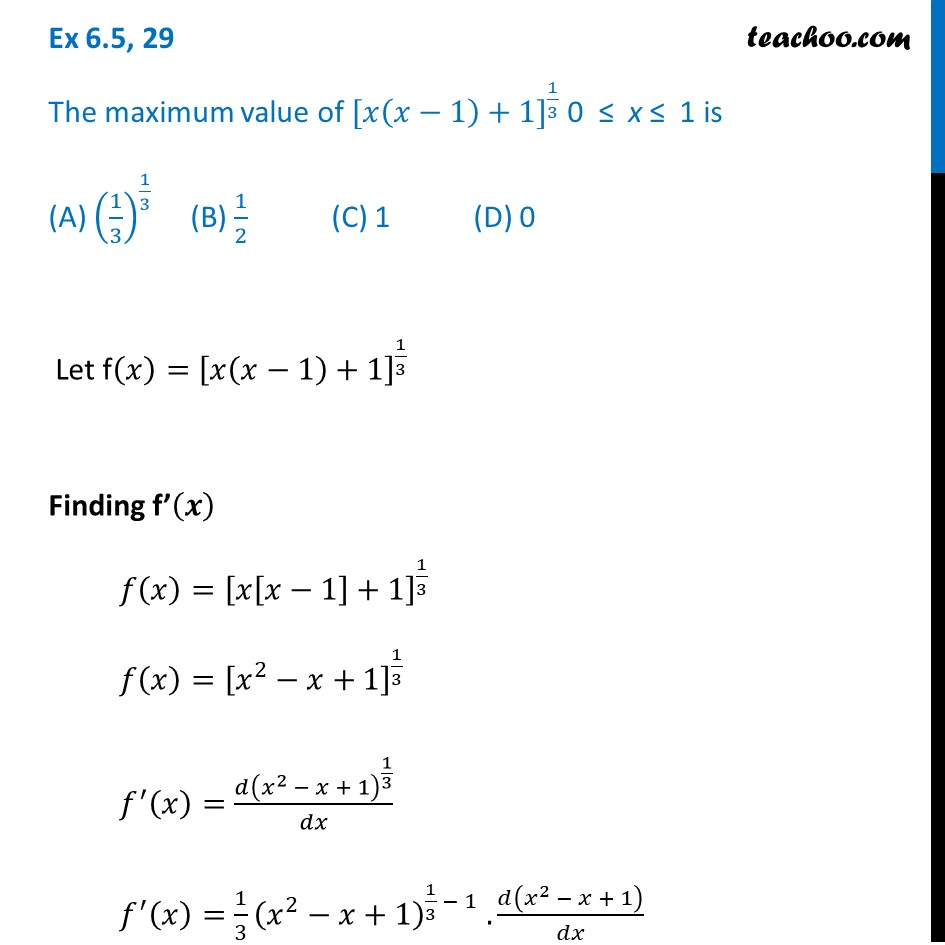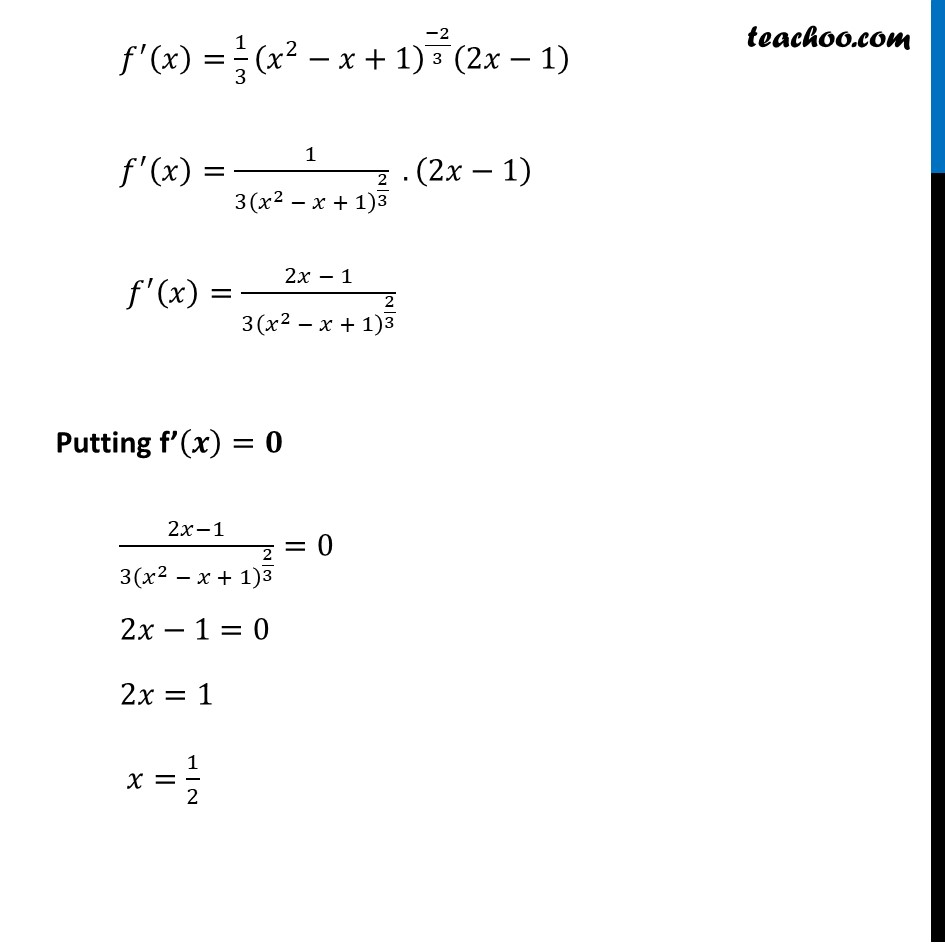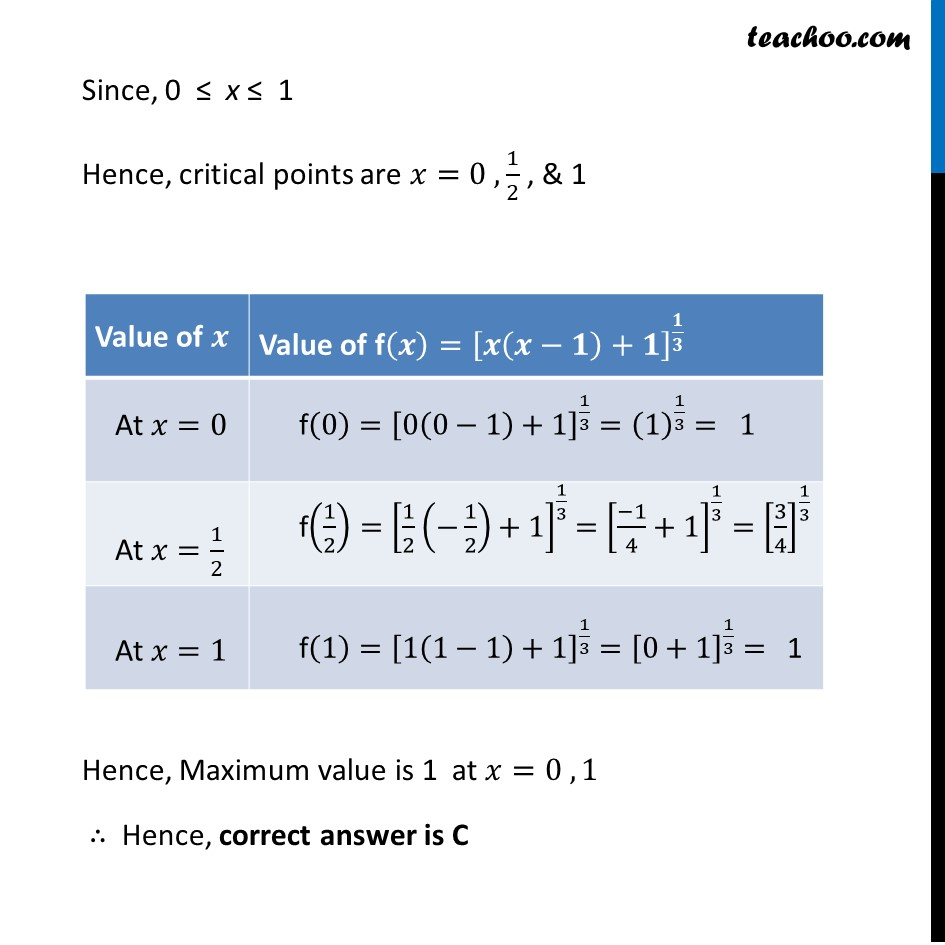1. Chapter 6 Class 12 Application of Derivatives
2. Serial order wise
3. Ex 6.5

Transcript

Ex 6.5, 29 The maximum value of 〖[𝑥(𝑥−1)+1]〗^(1/3) 0 ≤ x ≤ 1 is (A) (1/3)^(1/3) (B) 1/2 (C) 1 (D) 0 Let f(𝑥)=[𝑥(𝑥−1)+1]^(1/3) Finding f’(𝒙) 𝑓(𝑥)=[𝑥[𝑥−1]+1]^(1/3) 𝑓(𝑥)=[𝑥^2−𝑥+1]^(1/3) 𝑓^′ (𝑥)=(𝑑(𝑥^2 − 𝑥 + 1)^(1/3))/𝑑𝑥 𝑓^′ (𝑥)=1/3 (𝑥^2−𝑥+1)^(1/3 − 1) . 𝑑(𝑥^2 − 𝑥 + 1)/𝑑𝑥 𝑓^′ (𝑥)=1/3 (𝑥^2−𝑥+1)^((−2)/3) (2𝑥−1) 𝑓^′ (𝑥)=1/(3(𝑥^2 − 𝑥 + 1)^(2/3) ) .(2𝑥−1) 𝑓^′ (𝑥)=(2𝑥 − 1)/(3(𝑥^2 − 𝑥 + 1)^(2/3) ) Putting f’(𝒙)=𝟎 (2𝑥−1)/(3(𝑥^2 − 𝑥 + 1)^(2/3) )=0 2𝑥−1=0 2𝑥=1 𝑥=1/2 Since, 0 ≤ x ≤ 1 Hence, critical points are 𝑥=0 ,1/2 , & 1 Hence, Maximum value is 1 at 𝑥=0 , 1 ∴ Hence, correct answer is C

Ex 6.5